# Lions Weekly: December 1, 2021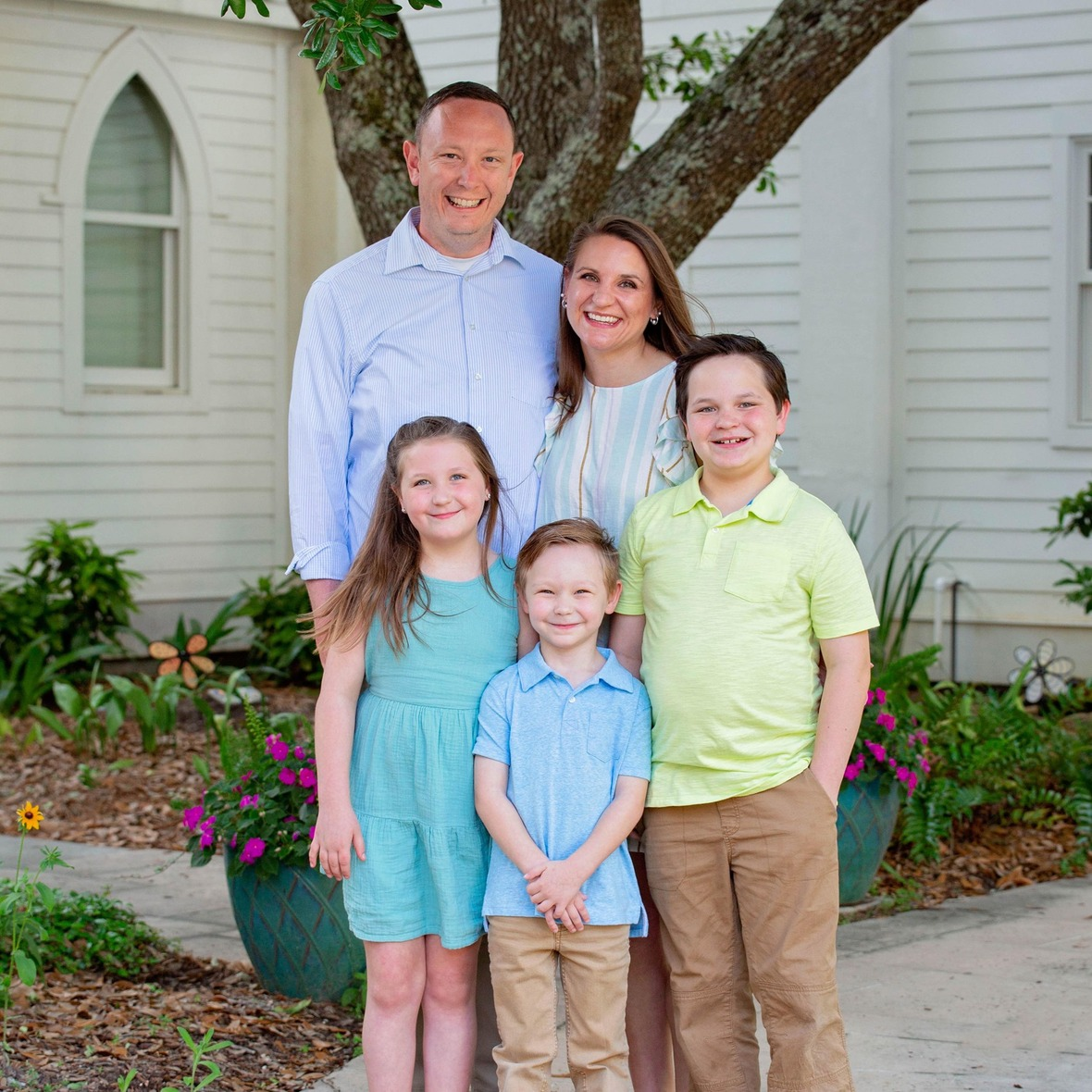# A Note From Mr. Sandefur

Lion Family,

First, I would like to thank all who so graciously gave yesterday as a part of National Giving Tuesday. Due to the generosity of those who gave, we were blessed to receive \$10,000 for our Legacy Fund, which will greatly bless our school community. We are humbled and grateful for the love and support from our TKA families. For those who I haven’t had the opportunity to discuss the details of our Legacy Fund with, I would like to take this opportunity to do so.

The “Legacy Fund” is an undesignated fund of financial development that allows flexibility in prioritizing campus needs. By giving to the “Legacy Fund,” stakeholders can make an immediate impact by allowing the highest priority needs to be addressed. Each calendar year, priorities will be listed for transparency, while also allowing funds to be used in the event of campus emergencies and urgent needs.

This year the Legacy Fund has already provided the following; furnishings (tables, benches, countertops, cooking equipment) for the Dr. Carroll Reece Reagan Pavilion, updated branding materials, new marketing and admissions communications and materials, Love Where We Live materials for the Seymour community, Student Life activities (homecoming, fellowships, residence life activities, etc.), and teacher blessings. Because of the faithful giving from supporters of The King’s Academy, the Legacy Fund will allow us to achieve greater things outside of regular tuition and fees that are collected.

I deeply appreciate your support of mission-minded, Christ-centered education here at The King’s Academy and am excited to celebrate the Christmas season with you. The information below contains a plethora of events where we can celebrate Christ in the coming days and I personally hope to see you soon.

In Christ Alone,

Jeremy Sandefur
President

 table div table+table+table+table div table{width:100%;padding:0}table div table+table+table+table div table img{width:96.23%;padding:0;float:none}table div table+table+table+table div table td{width:100%;padding:0 1.88% 18px}/* styles */# Save the Upcoming Dates!

As the Christmas season is approaching, we want to invite you to attend the many different Christmas celebrations happening on campus! Save the dates for the upcoming events listed below and celebrate the Christmas season with us at TKA!# TKA Instrumental Program

The TKA Middle and High school bands invite you to attend the Celebrate the Music of Christmas Concert on Thursday, December 2, at 6:30 p.m. in the Woody Auditorium.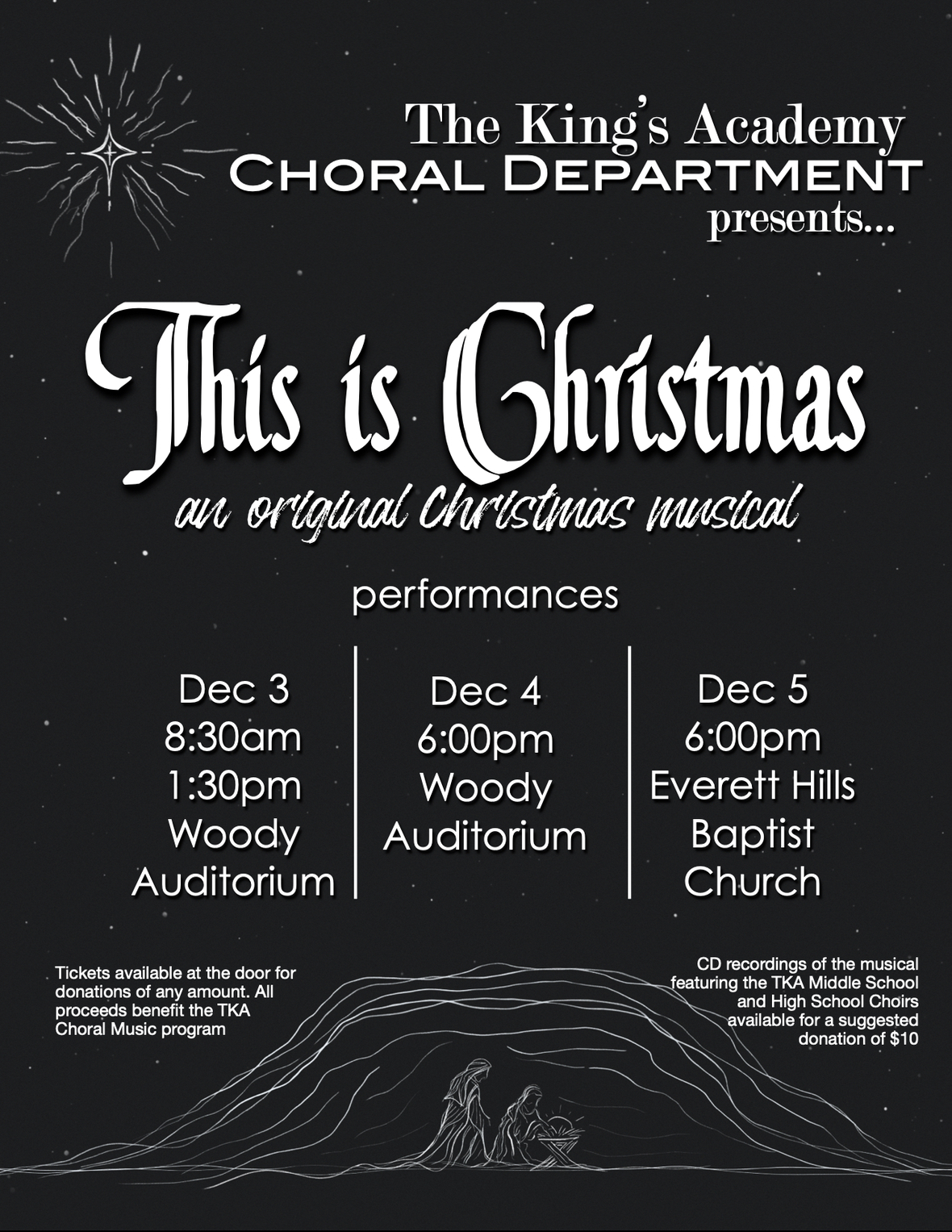# TKA Choral Department

Please mark your calendars and save the dates for our upcoming Christmas performances!

▪ December 3 at 8:30 a.m. and 1:30 p.m. in Woody Auditorium.
▪ December 4 at 6:00 p.m. in Woody Auditorium.
▪ December 5 at 6:00 p.m. at Everett Hills Baptist Church.
 ▪ December 3 at 8:30 a.m. and 1:30 p.m. in Woody Auditorium.
 ▪ December 4 at 6:00 p.m. in Woody Auditorium.
 ▪ December 5 at 6:00 p.m. at Everett Hills Baptist Church.

We look forward to celebrating This is Christmas with you!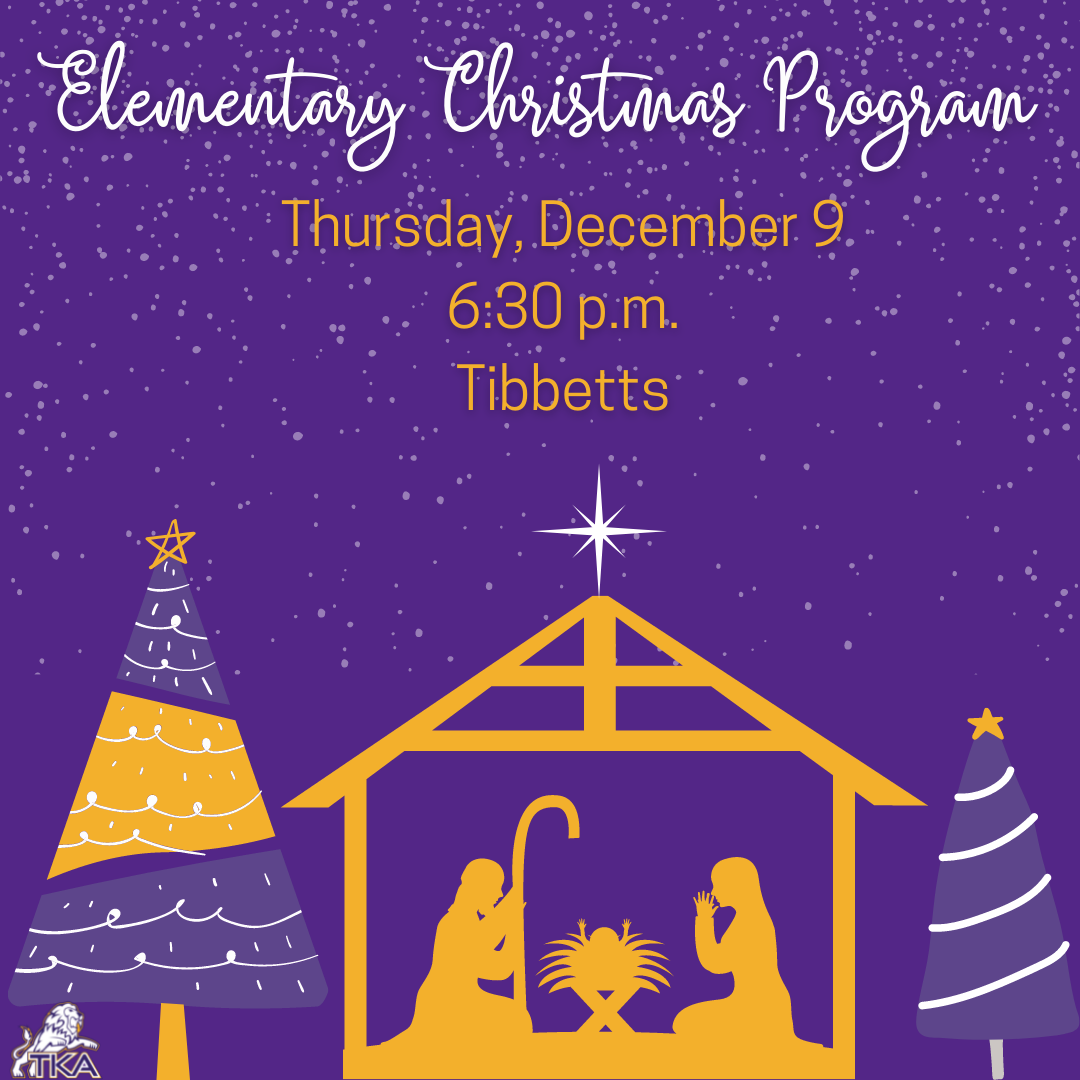# Elementary Christmas Program

Our elementary Christmas program will take place on Thursday, December 9, in Tibbetts. We are looking forward to celebrating the Christmas season with our loved ones through this program.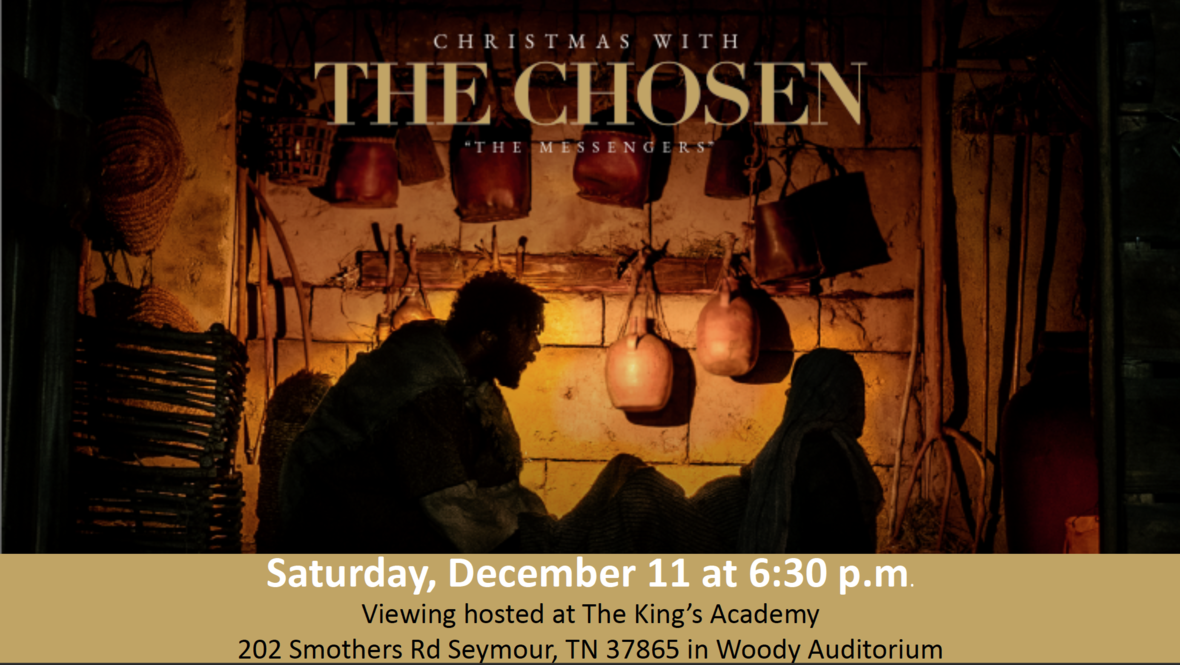Click the image to view The Chosen trailer

# Movie Showing

The King's Academy has been selected to host a viewing of "Christmas with The Chosen: The Messengers". To launch the Christmas season this December, The Chosen has created a special episode about the birth of Christ through the eyes of Mary and Joseph. You’ll also see an extraordinary lineup of musicians perform both new and classic Christmas songs from the set of The Chosen, including Phil Wickham, For King & Country, Brandon Lake, Maverick City Music, Cain, Matt Maher, Bryan & Katie Torwalt, Jordan Feliz, We The Kingdom, The Bonner Family, Leanna Crawford, Dawson Hollow, and One Voice Choir.

This is the perfect opportunity to bring friends and family, including those who haven’t seen The Chosen, for a unique Christmas experience.

Join us on Saturday, December 11 at 6:30 p.m. in Woody auditorium for this special event! Tickets for this event can be purchased by clicking here.

 table div table+table+table+table+table+table+table+table+table+table div table{width:100%;padding:0}table div table+table+table+table+table+table+table+table+table+table div table img{width:96.23%;padding:0;float:none}table div table+table+table+table+table+table+table+table+table+table div table td{width:100%;padding:0 1.88% 18px}/* styles */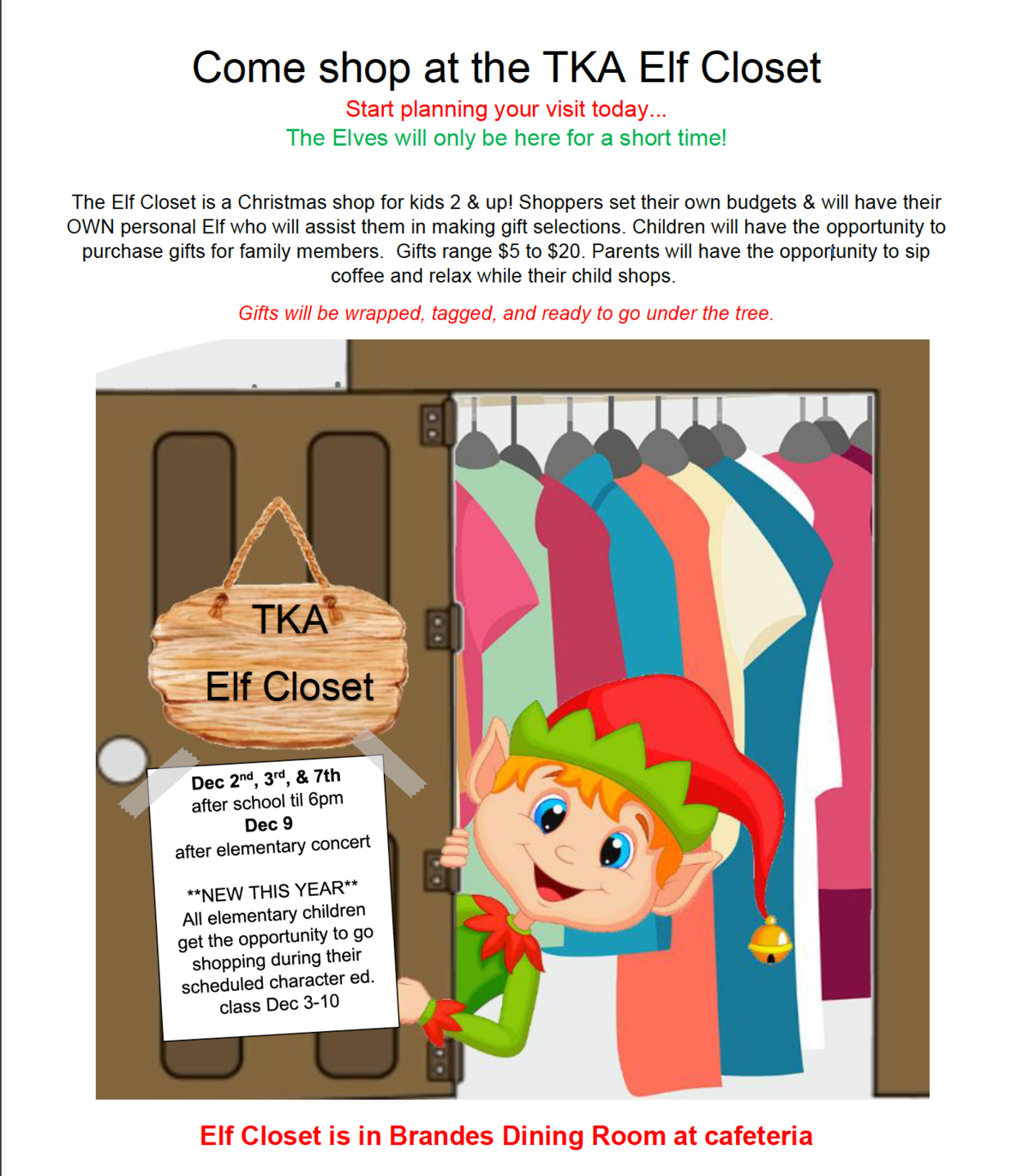# Elf Closet

Come shop at the TKA Elf Coset! The Elves will only be here for a short time.

The Elf Closet is a Christmas shop for kids 2 & up! Shoppers set their own budgets & will have their OWN personal Elf who will assist them in making gift selections. Children will have the opportunity to purchase gifts for family members. Gifts range \$5 to \$20. Parents will have the opportunity to sip coffee and relax while their child shops. Gifts will be wrapped, tagged, and ready to go under the tree.

We need elves to help the little shoppers(middle and high schoolers needed!!)!

For questions about the Elf Closet or to volunteer to help, contact Sarah Knight at 865-621-0741.

 table div table+table+table+table+table+table+table+table+table+table+table+table div table{width:100%;padding:0}table div table+table+table+table+table+table+table+table+table+table+table+table div table img{width:96.23%;padding:0;float:none}table div table+table+table+table+table+table+table+table+table+table+table+table div table td{width:100%;padding:0 1.88% 18px}/* styles */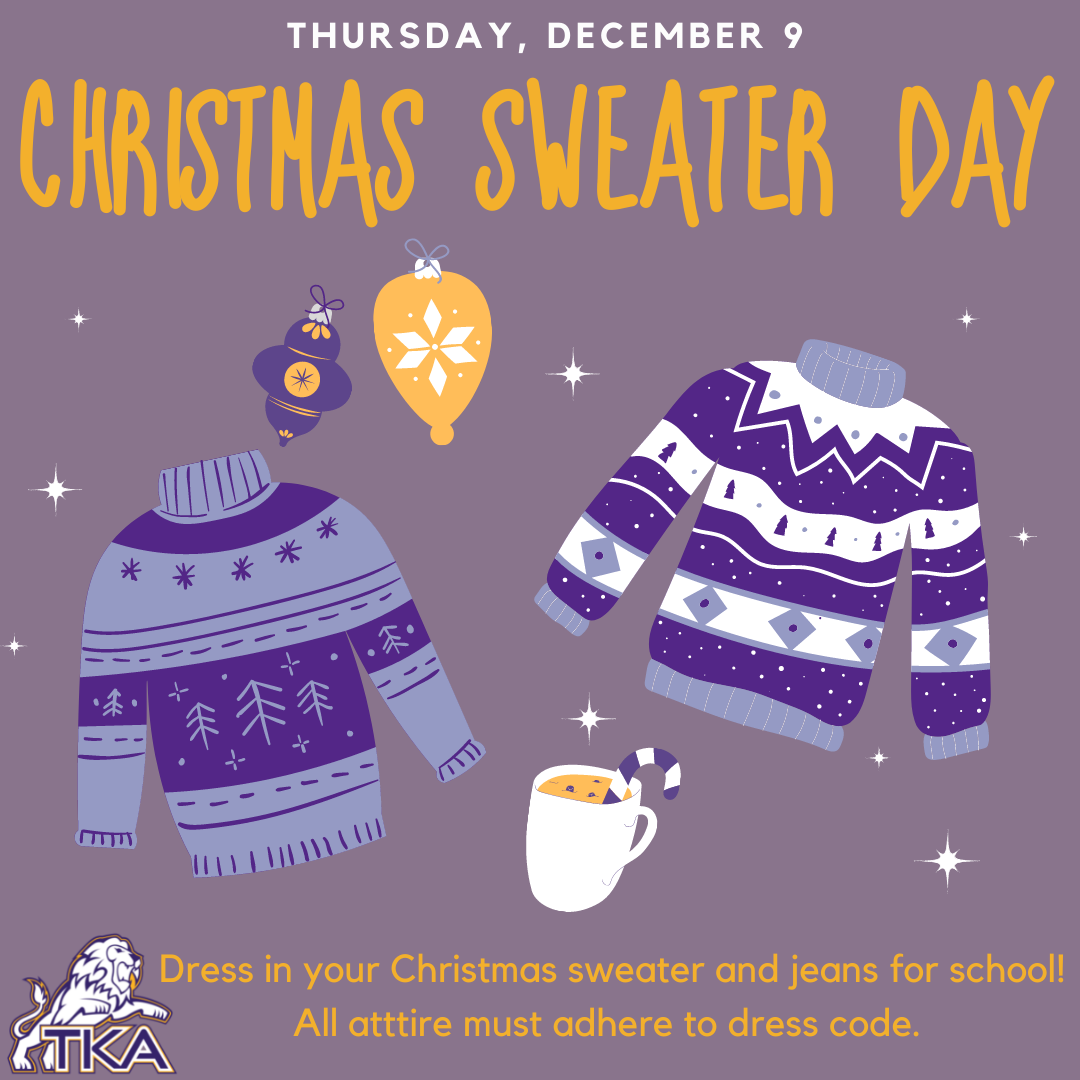# Christmas Sweater Day

On Thursday, December 9, students can dress in their favorite Christmas Sweater and jeans for school! There is no cost to participate in this day.

Remember, all attire must adhere to dress code.

 table div table+table+table+table+table+table+table+table+table+table+table+table+table+table div table{width:100%;padding:0}table div table+table+table+table+table+table+table+table+table+table+table+table+table+table div table img{width:96.23%;padding:0;float:none}table div table+table+table+table+table+table+table+table+table+table+table+table+table+table div table td{width:100%;padding:0 1.88% 18px}/* styles */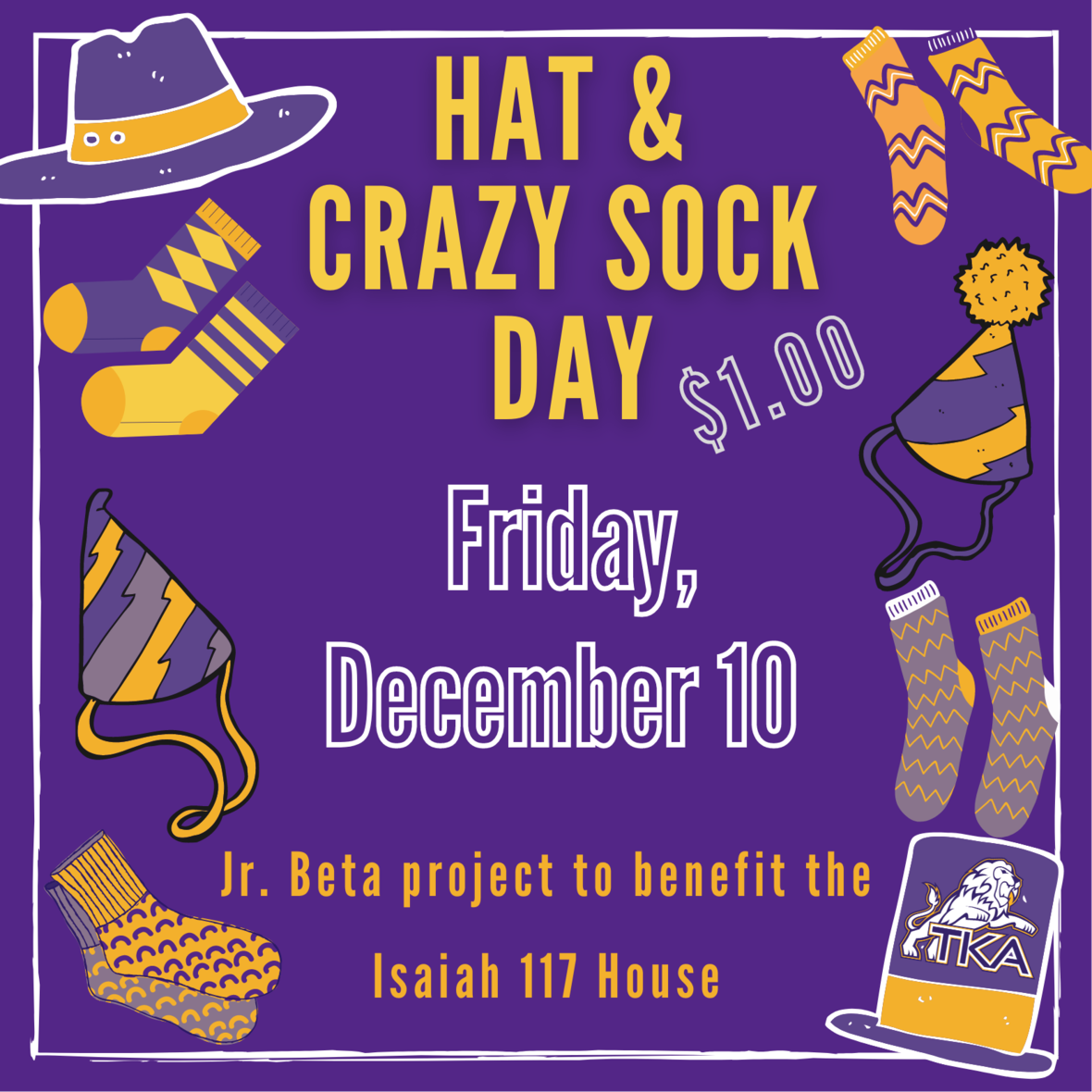# Hat and Crazy Sock Day

Jr. Beta is hosting a Hat and Crazy Sock Day to benefit the Isaiah 117 House.

Students can bring a \$1.00 (or more donation) to participate, OR students can bring a new toboggan to donate to the Isaiah 117 House. All financial and toboggan donations will be given directly to the Isaiah 117 House.

 table div table+table+table+table+table+table+table+table+table+table+table+table+table+table+table+table div table{width:100%;padding:0}table div table+table+table+table+table+table+table+table+table+table+table+table+table+table+table+table div table img{width:96.23%;padding:0;float:none}table div table+table+table+table+table+table+table+table+table+table+table+table+table+table+table+table div table td{width:100%;padding:0 1.88% 18px}/* styles */# Upcoming Weekly Sporting Events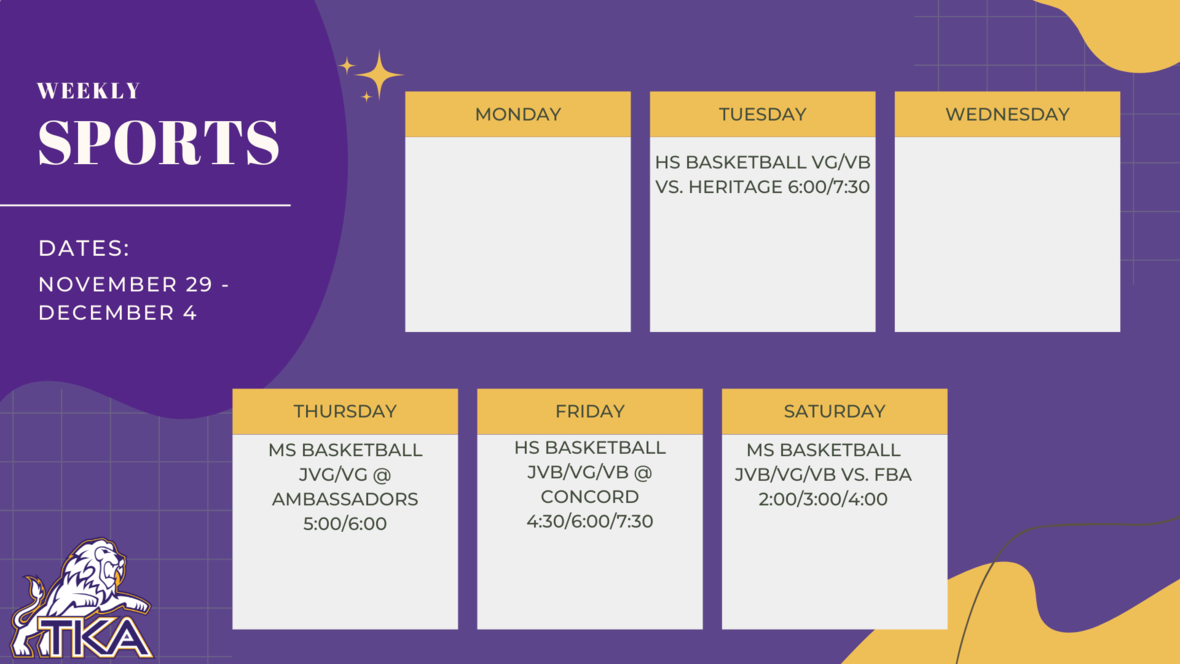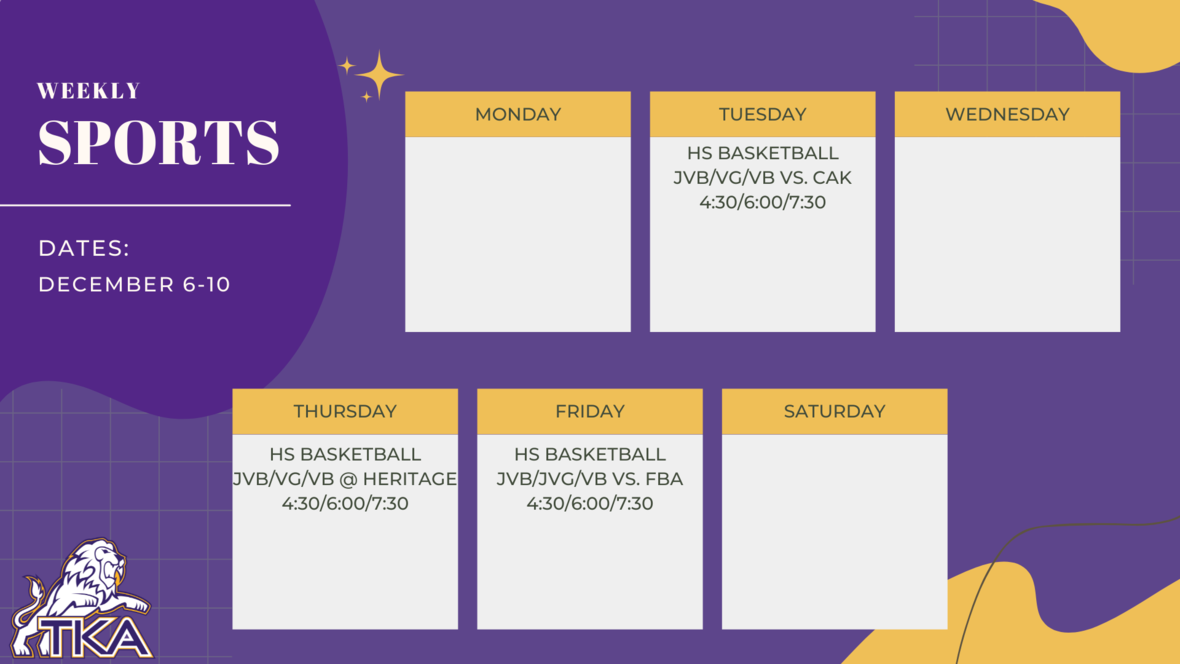table div table+table+table+table+table+table+table+table+table+table+table+table+table+table+table+table+table+table+table+table div table{width:100%;padding:0}table div table+table+table+table+table+table+table+table+table+table+table+table+table+table+table+table+table+table+table+table div table img{width:96.23%;padding:0;float:none}table div table+table+table+table+table+table+table+table+table+table+table+table+table+table+table+table+table+table+table+table div table td{width:100%;padding:0 1.88% 18px}/* styles */# Elementary Clubs

All fall elementary clubs will end next week by Friday, December 10.

For those who would like to participate in elementary clubs for the spring semester, sign up forms and information will be shared in January.

 table div table+table+table+table+table+table+table+table+table+table+table+table+table+table+table+table+table+table+table+table+table+table div table{width:100%;padding:0}table div table+table+table+table+table+table+table+table+table+table+table+table+table+table+table+table+table+table+table+table+table+table div table img{width:96.23%;padding:0;float:none}table div table+table+table+table+table+table+table+table+table+table+table+table+table+table+table+table+table+table+table+table+table+table div table td{width:100%;padding:0 1.88% 18px}/* styles */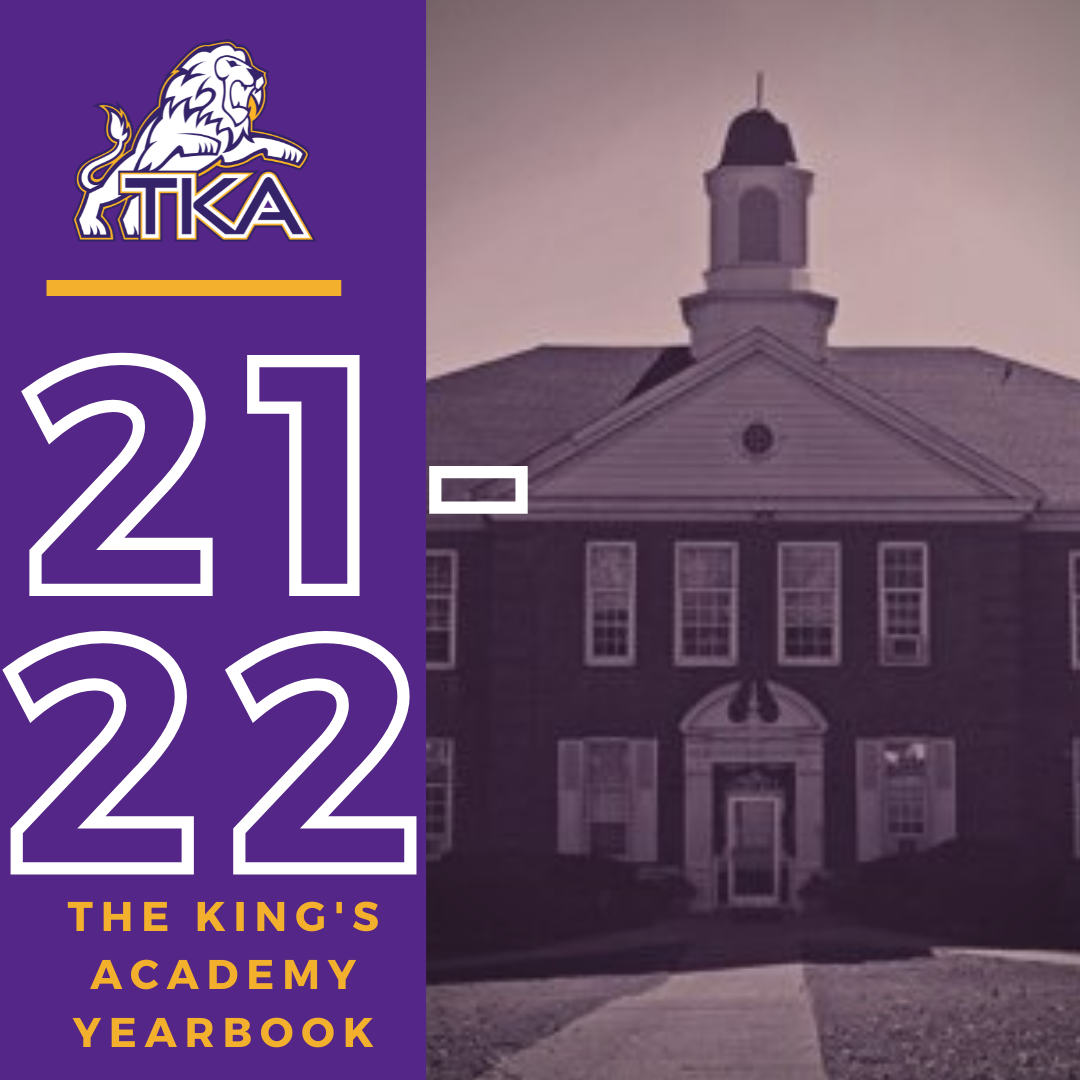# 2021-2022 Yearbook

The price for the 2021-2022 TKA yearbook is \$55. All grades, K3-12 will have the same yearbook. The slight price increase is because we are only going to have one larger all-school book.

All yearbook orders can be placed online--here is the link.

Senior Tributes and Business ads are available for purchase at the bottom of the link listed here.

For any questions regarding yearbooks, email Mr. Josh Smith at jsmith@thekingsacademy.net.

 table div table+table+table+table+table+table+table+table+table+table+table+table+table+table+table+table+table+table+table+table+table+table+table+table div table{width:100%;padding:0}table div table+table+table+table+table+table+table+table+table+table+table+table+table+table+table+table+table+table+table+table+table+table+table+table div table img{width:96.23%;padding:0;float:none}table div table+table+table+table+table+table+table+table+table+table+table+table+table+table+table+table+table+table+table+table+table+table+table+table div table td{width:100%;padding:0 1.88% 18px}/* styles */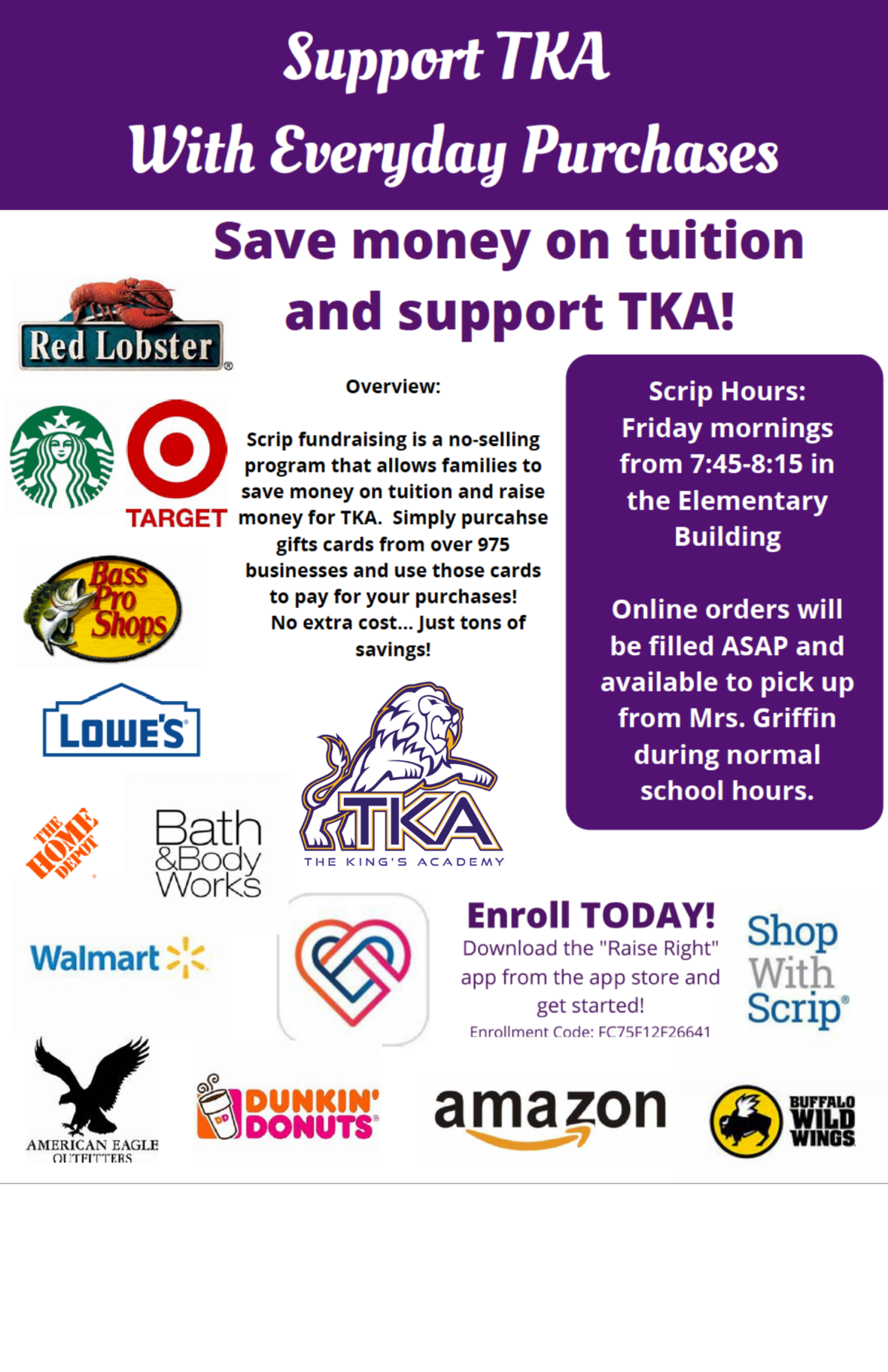# TKA Scrip

As the holidays are approaching and Christmas shopping is beginning, don't forget about our TKA Scrip program!

Scrip is a no-selling program that allows families to save money on tuition and raise money for TKA by purchasing gift cards from over 975 businesses! Gift cards can be purchased in person each Friday morning from 7:45-8:15, or orders can be made online by clicking here. The enrollment code is: FC75F12F26641

Thank you for taking time to support our TKA Scrip program!

 table div table+table+table+table+table+table+table+table+table+table+table+table+table+table+table+table+table+table+table+table+table+table+table+table+table+table div table{width:100%;padding:0}table div table+table+table+table+table+table+table+table+table+table+table+table+table+table+table+table+table+table+table+table+table+table+table+table+table+table div table img{width:96.23%;padding:0;float:none}table div table+table+table+table+table+table+table+table+table+table+table+table+table+table+table+table+table+table+table+table+table+table+table+table+table+table div table td{width:100%;padding:0 1.88% 18px}/* styles */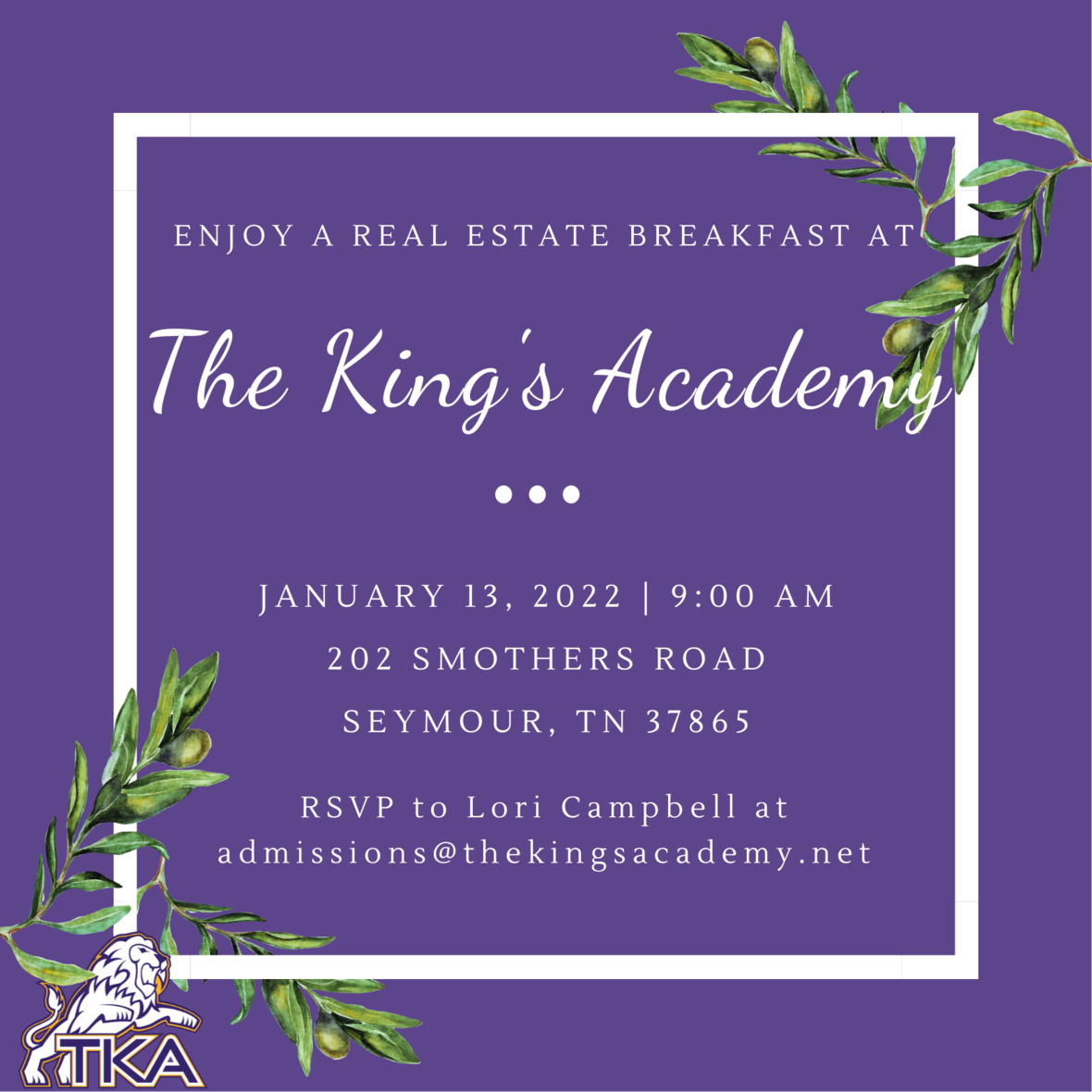# Real Estate Breakfast

Seymour and the surrounding area has been growing quickly, with many new prospective families moving into our community. In an effort to share the story of TKA with our neighbors, we would like to invite local realtors and real estate agents to breakfast on Thursday, January 13, at 9:00 in the Brandes Cafeteria. Please RSVP by January 5 to reserve your seat and informational materials. Click here to RSVP.

 table div table+table+table+table+table+table+table+table+table+table+table+table+table+table+table+table+table+table+table+table+table+table+table+table+table+table+table+table div table{width:100%;padding:0}table div table+table+table+table+table+table+table+table+table+table+table+table+table+table+table+table+table+table+table+table+table+table+table+table+table+table+table+table div table img{width:96.23%;padding:0;float:none}table div table+table+table+table+table+table+table+table+table+table+table+table+table+table+table+table+table+table+table+table+table+table+table+table+table+table+table+table div table td{width:100%;padding:0 1.88% 18px}/* styles */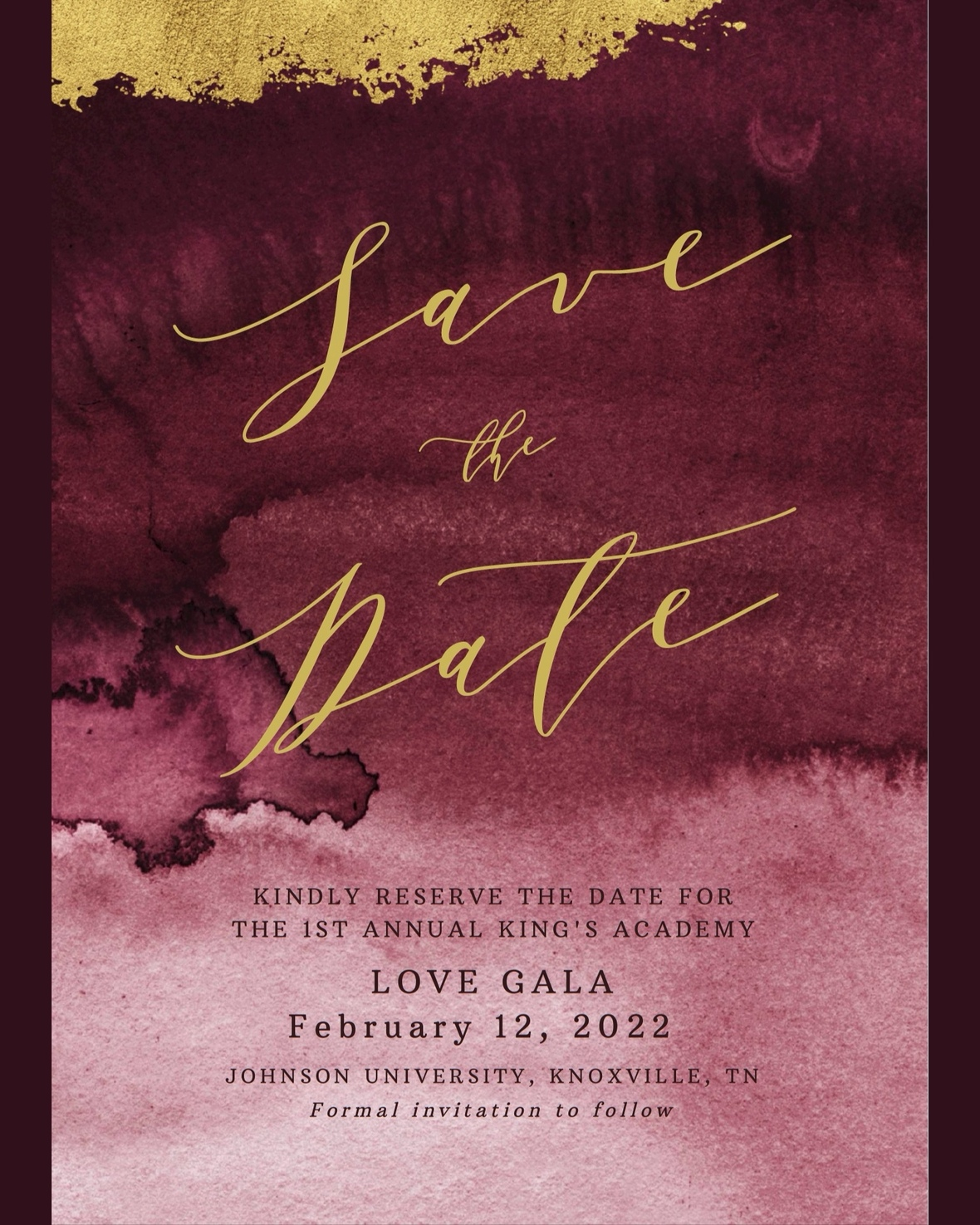# Love Gala

Save the date for the first annual Love Gala hosted by The King's Academy. The Love Gala will be on February 12, 2022, at Johnson University in Knoxville, TN. Plan on joining us for a night of fellowship, an exciting auction with great items, and a time to honor our alumni, teachers, and those who have left a legacy at TKA.

Each ticket purchased for the event will have an opportunity to win FREE tuition for one student for the 2022-23 academic year. Additional tuition remission opportunity tickets are available for purchase at the door for \$25 per opportunity. All opportunity drawing tickets are Non-Transferable. Only the student drawn is eligible for tuition remission. Must be present to receive tuition remission.

 table div table+table+table+table+table+table+table+table+table+table+table+table+table+table+table+table+table+table+table+table+table+table+table+table+table+table+table+table+table+table div table{width:100%;padding:0}table div table+table+table+table+table+table+table+table+table+table+table+table+table+table+table+table+table+table+table+table+table+table+table+table+table+table+table+table+table+table div table img{width:96.23%;padding:0;float:none}table div table+table+table+table+table+table+table+table+table+table+table+table+table+table+table+table+table+table+table+table+table+table+table+table+table+table+table+table+table+table div table td{width:100%;padding:0 1.88% 18px}/* styles *//* styles */
 table div table+table+table+table+table+table+table+table+table+table+table+table+table+table+table+table+table+table+table+table+table+table+table+table+table+table+table+table+table+table+table+table+table div table{width:100%;padding:0}table div table+table+table+table+table+table+table+table+table+table+table+table+table+table+table+table+table+table+table+table+table+table+table+table+table+table+table+table+table+table+table+table+table div table img{width:96.23%;padding:0;float:none}table div table+table+table+table+table+table+table+table+table+table+table+table+table+table+table+table+table+table+table+table+table+table+table+table+table+table+table+table+table+table+table+table+table div table td{width:100%;padding:0 1.88% 18px}/* styles */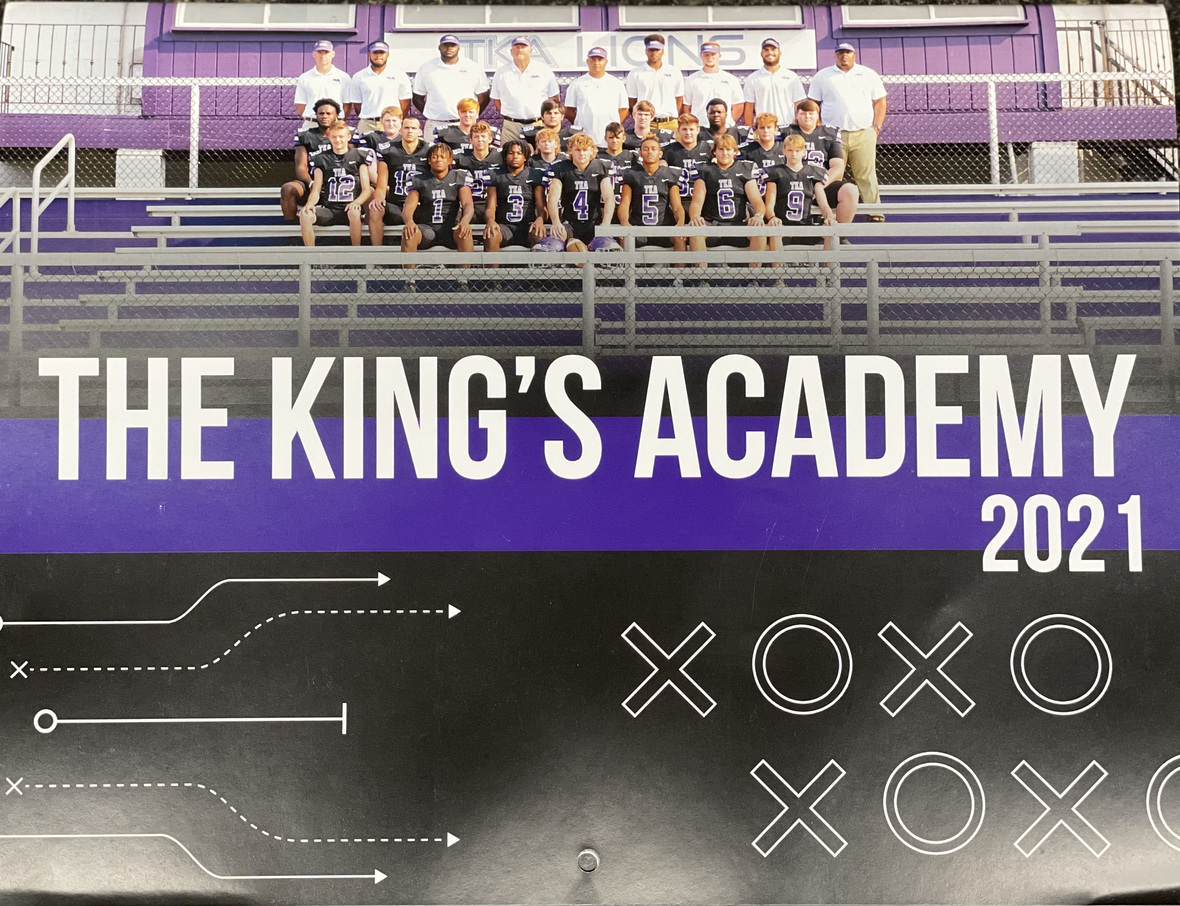# 2021-2022 Football Calendar

This year's football calendars are for sale for \$10! Stop by the school office to pick up your copy today!

 table div table+table+table+table+table+table+table+table+table+table+table+table+table+table+table+table+table+table+table+table+table+table+table+table+table+table+table+table+table+table+table+table+table+table+table div table{width:100%;padding:0}table div table+table+table+table+table+table+table+table+table+table+table+table+table+table+table+table+table+table+table+table+table+table+table+table+table+table+table+table+table+table+table+table+table+table+table div table img{width:96.23%;padding:0;float:none}table div table+table+table+table+table+table+table+table+table+table+table+table+table+table+table+table+table+table+table+table+table+table+table+table+table+table+table+table+table+table+table+table+table+table+table div table td{width:100%;padding:0 1.88% 18px}/* styles */table div table+table+table+table+table+table+table+table+table+table+table+table+table+table+table+table+table+table+table+table+table+table+table+table+table+table+table+table+table+table+table+table+table+table+table+table+table div table{width:100%;padding:0}table div table+table+table+table+table+table+table+table+table+table+table+table+table+table+table+table+table+table+table+table+table+table+table+table+table+table+table+table+table+table+table+table+table+table+table+table+table div table img{width:96.23%;padding:0;float:none}table div table+table+table+table+table+table+table+table+table+table+table+table+table+table+table+table+table+table+table+table+table+table+table+table+table+table+table+table+table+table+table+table+table+table+table+table+table div table td{width:100%;padding:0 1.88% 18px}/* styles */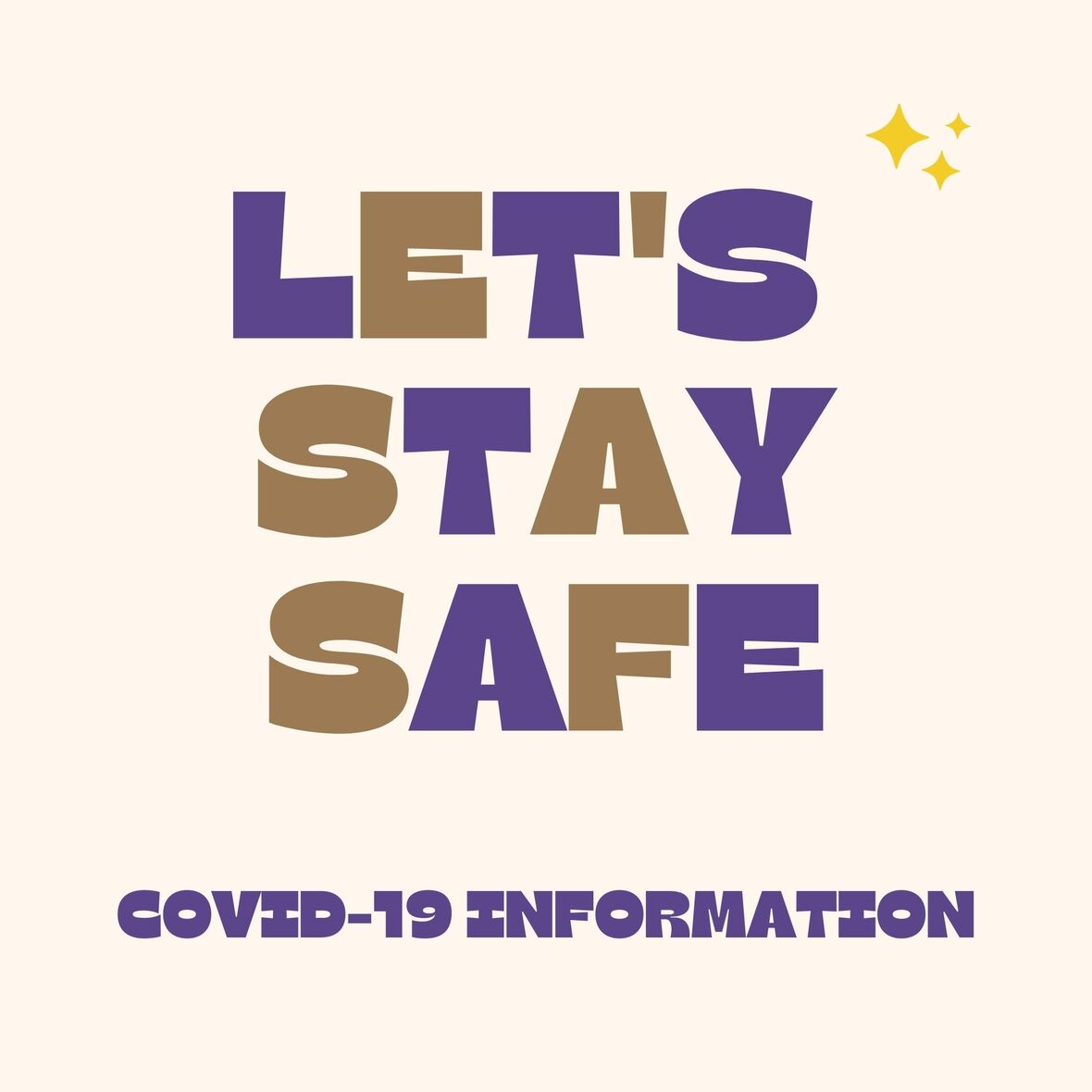# Covid-19 Procedures

While we are excited to offer in-person education, we must maintain a safe learning environment for all students, faculty/staff, and additional stakeholders.

To do so, we will be working closely with the Sevier County Health Department regarding Covid-19 protocols.

 table div table+table+table+table+table+table+table+table+table+table+table+table+table+table+table+table+table+table+table+table+table+table+table+table+table+table+table+table+table+table+table+table+table+table+table+table+table+table+table div table{width:100%;padding:0}table div table+table+table+table+table+table+table+table+table+table+table+table+table+table+table+table+table+table+table+table+table+table+table+table+table+table+table+table+table+table+table+table+table+table+table+table+table+table+table div table img{width:96.23%;padding:0;float:none}table div table+table+table+table+table+table+table+table+table+table+table+table+table+table+table+table+table+table+table+table+table+table+table+table+table+table+table+table+table+table+table+table+table+table+table+table+table+table+table div table td{width:100%;padding:0 1.88% 18px}/* styles */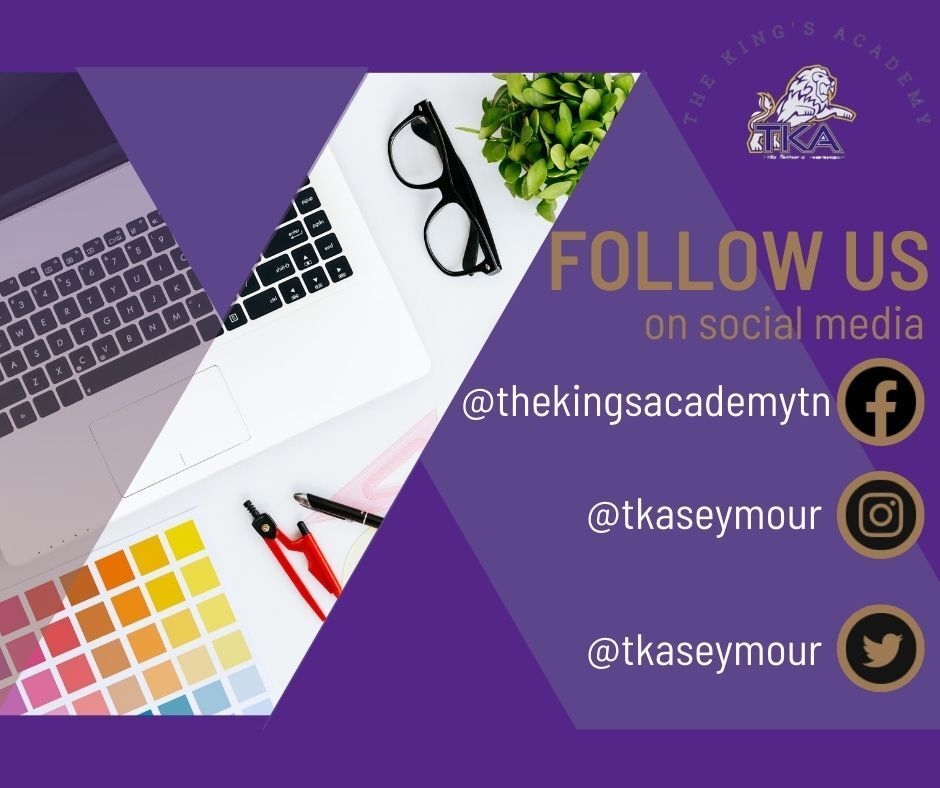table div table+table+table+table+table+table+table+table+table+table+table+table+table+table+table+table+table+table+table+table+table+table+table+table+table+table+table+table+table+table+table+table+table+table+table+table+table+table+table+table+table div table{width:100%;padding:0}table div table+table+table+table+table+table+table+table+table+table+table+table+table+table+table+table+table+table+table+table+table+table+table+table+table+table+table+table+table+table+table+table+table+table+table+table+table+table+table+table+table div table img{width:96.23%;padding:0;float:none}table div table+table+table+table+table+table+table+table+table+table+table+table+table+table+table+table+table+table+table+table+table+table+table+table+table+table+table+table+table+table+table+table+table+table+table+table+table+table+table+table+table div table td{width:100%;padding:0 1.88% 18px}/* styles */